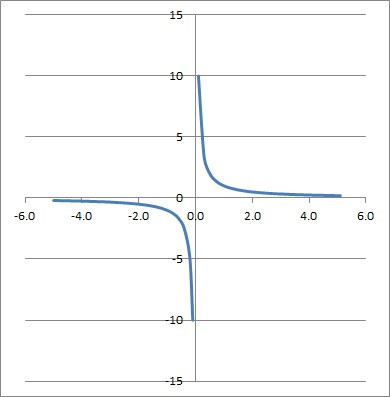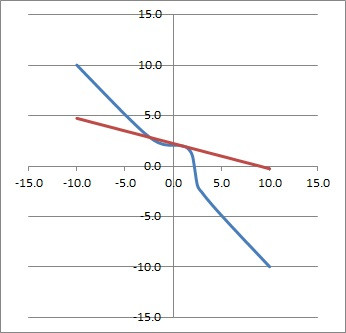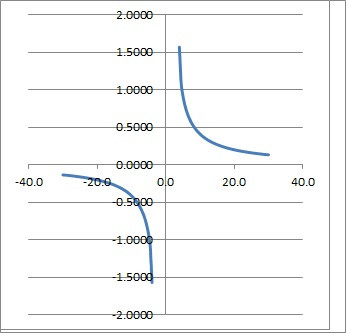# What Is Implicit Differentiation? (3 Key Ideas To Know)

Implicit differentiation is often used in calculus when we have a function where it is difficult to isolate one of the variables.

So, what is implicit differentiation?  Implicit differentiation uses the chain rule to find the derivative of an implicit function. In implicit differentiation with respect to x, the derivative of y is y’ or dy/dx. After implicit differentiation, we isolate y’ to find a derivative in terms of the variables in the implicit function.

In some cases, we can take the second, third, or higher order derivatives with implicit differentiation.

In this article, we’ll talk about implicit differentiation and look at some examples to show how it works.

Let’s get started.

## What Is Implicit Differentiation?

Implicit differentiation is a method that uses the chain rule to find the derivative of an implicit function.

In implicit differentiation with respect to x, the derivative of y is y’ or dy/dx (the derivative of x is 1). The normal rules of derivatives (power rule, product rule, etc.) still apply.

After implicit differentiation, we isolate y’ to find a derivative in terms of the variables in the implicit function.

### Why Do We Use Implicit Differentiation?

We use implicit differentiation when it is impossible or impractical to isolate the dependent variable.

For example:

• √(2x + y) + 3√(x + 2y) = 1
• exy + x + y = 3x + 4y + 5

In these cases, we can use implicit differentiation to find the slope of a function at a given point. This helps us to figure out what the behavior looks like, even if we cannot isolate a particular variable.

### Implicit Differentiation Steps

The steps for implicit differentiation vary depending on the function and expressions involved. For example, for xy = 1, we would use the product rule, while for xsin(y) = ysin(x) we would use the rule for derivatives of sine and cosine.

However, it does help to look at some examples to see how this method works.

### How To Do Implicit Differentiation (Examples)

To do implicit differentiation, we start by taking the derivative of both sides of the equation (usually with respect to x).

Here are some examples.

#### Example 1: Implicit Differentiation (Product Of Two Variables)

Let’s say we have the function xy = 1.

We will use implicit differentiation to find y’ or dy/dx.

First, take the derivative of both sides with respect to x:

• d/dx[xy] = d/dx
• d/dx[xy] = 0  [derivative of any constant with respect to any variable is 0]
• (1)(y) + (x)(y’) = 0  [product rule used on the left side; dy/dx = y’]
• y + xy’ = 0
• xy’ = -y
• y’ = -y/x

So, y’ = dy/dx = -y/x.

The original equation is xy = 1, which we can solve as y = 1/x. Using this in the y’ equation, we get:

• y’ = -y/x
• y’ = -(1/x)/x  [y = 1/x]
• y’ = -1/x2

We can verify this by taking the derivative of y with respect to x and using the power rule for derivatives negative exponents:

• y = 1/x
• y = x-1
• dy/dx = -1x-2
• y’ = -1/x2

The derivative y’ = -1/x2 is always negative, which makes sense based on the graph of the reciprocal function y = 1/x (or xy = 1) below.This is the graph of xy = 1, or y = 1/x (the reciprocal function). We can use implicit differentiation to find y’ = -1/x2, meaning the derivative is always negative (the function is always decreasing).

#### Example 2: Implicit Differentiation (Higher Powers)

Let’s say we have the function x3 + y3 = 9.

We will use implicit differentiation to find y’ or dy/dx.

First, take the derivative of both sides with respect to x:

• d/dx[x3 + y3] = d/dx
• d/dx[x3 + y3] = 0  [derivative of any constant with respect to any variable is zero]
• 3x2 + 3y2y’ = 0
• 3y2y’ = -3x2
• y2y’ = -x2
• y’ = -x2/y2

As in the example above, this derivative is always negative (except when zero, whenever x = 0).

We can use this derivative dy/dx = y’ = -x2/y2 to find the slope of the function x3 + y3 = 9 at a given point.

For instance, at the point (1, 2), where x = 1 and y = 2, we get a slope of:

• y’ = -x2/y2
• y’ = -(1)2/(2)2
• y’ = -1/4

So, the slope at the point (1, 2) is dy/dx = y’ = -1/4.

In fact, we can the tangent line y = mx + b at this point if we solve for b:

• y = mx + b
• 2 = (-1/4)(1) + b
• 2 = -1/4 + b
• 9/4 = b

So, the tangent line is given by the equation y = -0.25x + 2.25.  You can see this tangent line of the graph of x3 + y3 = 9 below:This is the graph of x3 + y3 = 9 (blue curve). We can use implicit differentiation to find y’ = -x2/y2, meaning the derivative is always negative or zero (the function is always decreasing). The behavior approaches that of the line y = -x as x approaches infinity (and y’ approaches -1). The red line is the tangent line at the point (1, 2).

#### Example 3: Implicit Differentiation (Trigonometric Functions)

Let’s say we have the function sin(xy) + cos(xy) = 5x + 2

We will use implicit differentiation to find y’ or dy/dx.

First, take the derivative of both sides with respect to x:

• d/dx[sin(xy) + cos(xy)] = d/dx[5x + 2]
• d/dx[sin(xy) + cos(xy)] = 5
• cos(xy)*d/dx[xy] + -sin(xy)*d/dx[xy] = 5
• d/dx[xy](cos(xy) – sin(xy)) = 5
• (y + xy’)(cos(xy) – sin(xy)) = 5  [d/dx[xy] = y + xy’]
• y + xy’ = 5/(cos(xy) – sin(xy))
• xy’ = [5/(cos(xy) – sin(xy))] – y
• y’ = [5/x(cos(xy) – sin(xy))] – xy

#### Example 5: Implicit Differentiation (Exponential Functions)

Let’s say we have the function ex + ey = x2 + y2 + 2

We will use implicit differentiation to find y’ or dy/dx.

First, take the derivative of both sides with respect to x:

• d/dx[ex + ey] = d/dx[x2 + y2 + 2]
• d/dx[ex + ey] = 2x + 2yy’ + 0
• ex + ey*y’ = 2x + 2yy’ + 0
• ey*y’ – 2yy’ = 2x – ex  [isolate y’ on left side of equation]
• y’[ey – 2y] = 2x – ex
• y’ = (2x – ex) / (ey – 2y)

At the point (0, 0), we can find the derivative y’:

• y’ = (2x – ex) / (ey – 2y)
• y’ = (2(0) – e0) / (e0 – 2(0))
• y’ = (0 – 1) / (1 – 0)
• y’ = – 1 / 1
• y’ = -1

So, the slope is y’ = -1 at the point (0, 0).

### How To Find The Second Derivative With Implicit Differentiation

We can also find the second derivative with implicit differentiation. This just requires taking the derivative twice.

#### Example: Using Implicit Differentiation To Find The Second Derivative

Let’s say we have the function xsin(y) = 4 (graph shown below).The graph of xsin(y) = 4. It is undefined for -4 < x < 4, since 4/x would be greater than 1 or less than -1, and sin(y) is between -1 and 1.

We will use implicit differentiation twice to find y’’ or d2y /dx2.

First, take the derivative of both sides with respect to x:

• d/dx[xsin(y)] = d/dx
• d/dx[xsin(y)] = 0
• (1)sin(y) + xcos(y)*y’ = 0
• xy’cos(y) = -sin(y)
• y’ = -sin(y)/xcos(y)
• y’ = -tan(y)/x

Now, we take the derivative of both sides of the above equation with respect to x:

• d/dx[y’] = d/dx[-tan(y)/x]
• y’’ = d/dx[-tan(y)/x]
• y’’ = -d/dx[tan(y)/x]
• y’’ = -[(sec2(y)*y’*x – tan(y)*1]/x2
• y’’ = -[(sec2(y)*(-tan(y)/x)*x – tan(y)*1]/x2  [y’ = -tan(y)/x]
• y’’ = -[(sec2(y)*(-tan(y)) – tan(y)]/x2
• y’’ = -[-tan(y)(sec2(y) + 1)]/x2
• y’’ = [tan(y)(sec2(y) + 1)]/x2

## Conclusion

Now you know what implicit differentiation is and how to do it.